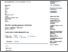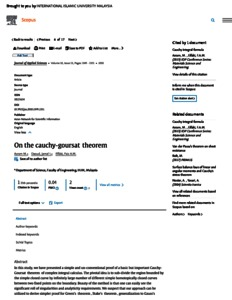# On the Cauchy-Goursat theorem

Azram, Mohammad and Daoud, Jamal Ibrahim and Elfaki, Faiz Ahmed Mohamed (2010) On the Cauchy-Goursat theorem. Journal of Applied Sciences, 10 (13). pp. 1349-1351. ISSN 1812-5654 E-ISSN 1812-5662PDF (On the cauchy-goursat) - Published Version
Restricted to Repository staff onlyPreview
PDF (SCOPUS) - Supplemental Material

## Abstract

Abstract: In this study, we have presented a simple and un-conventional proof of a basic but important Cauchy-Goursat theorem of complex integral calculus. The pivotal idea is to sub-divide the region bounded by the simple closed curve by infinitely large number of different simple homotopically closed curves between two fixed points on the boundary. Beauty of the method is that one can easily see the significant roll of singularities and analyticity requirements. We suspect that our approach can be utilized to derive simpler proof for Green ’s theorem, Stoke ’s theorem, generalization to Gauss ’s divergence theorem, extension of Cauchy-Goursat theorem to multiply connected regions, critical study of the affects of singularities over a general field with a general domain and a simpler approach for complex integration such as Cauchy integral formula, residue theorem etc. Avoiding topological and rigor mathematical requirements, we have sub-divided the region bounded by the simple closed curve by a large number of different simple closed curves between two fixed points on the boundary and have introduced: where path of integration is from p to q along ci and for I = 0, 1, 2, ..., n. Line integral along the boundary of the domain was evaluated via ( ∂f/ ∂z). Commutation between integration, δ-operation and d-operation was established. Using the vector interpretation of complex number, the area ds of a small parallelogram was established as . Finally, using Cauchy-Riemann equations we have established the well celebrated Cauchy-Goursat theorem, i.e., if a function f(z) is analytic inside and on a simple closed curve c then

Item Type: Article (Journal) 3127/1769 Cauchy-Goursat theorem, analytic function, simple closed curve, singular points, Cauchy-Riemann equations Q Science > QA Mathematics Kulliyyah of Engineering > Department of Science Prof Mohammad Azram 09 Sep 2011 09:04 01 Aug 2022 10:20 http://irep.iium.edu.my/id/eprint/1769View Item